fcm_fc模拟器 - CSDN
• 模糊C均值（Fuzzy C-means）算法简称FCM算法，是一种基于目标函数的模糊聚类算法，主要用于数据的聚类分析。理论成熟，应用广泛，是一种优秀的聚类算法。本文关于FCM算法的一些原理推导部分介绍等参考下...
【之前】 该文的pdf清晰版已被整理上传，方便保存学习，下载地址：

（一）原理部分

模糊C均值（Fuzzy C-means）算法简称FCM算法，是一种基于目标函数的模糊聚类算法，主要用于数据的聚类分析。理论成熟，应用广泛，是一种优秀的聚类算法。本文关于FCM算法的一些原理推导部分介绍等参考下面视频，加上自己的理解以文字的形式呈现出来，视频参考如下，比较长，看不懂的可以再去看看：

FCM原理介绍

FCM分析1
FCM分析2
FCM分析3

首先介绍一下模糊这个概念，所谓模糊就是不确定，确定性的东西是什么那就是什么，而不确定性的东西就说很像什么。比如说把20岁作为年轻不年轻的标准，那么一个人21岁按照确定性的划分就属于不年轻，而我们印象中的观念是21岁也很年轻，这个时候可以模糊一下，认为21岁有0.9分像年轻，有0.1分像不年轻，这里0.9与0.1不是概率，而是一种相似的程度，把这种一个样本属于结果的这种相似的程度称为样本的隶属度，一般用u表示，表示一个样本相似于不同结果的一个程度指标。

基于此，假定数据集为X，如果把这些数据划分成c类的话，那么对应的就有c个类中心为C，每个样本j属于某一类i的隶属度为uij${u}_{ij}$$u_{ij}$，那么定义一个FCM目标函数（1）及其约束条件（2）如下所示：

J=∑i=1c∑j=1numij||xj−ci||2(1)$\begin{array}{}\text{(1)}& J=\sum _{i=1}^{c}\sum _{j=1}^{n}{u}_{ij}^{m}||{x}_{j}-{c}_{i}|{|}^{2}\end{array}$J=\sum\limits_{i=1}^{c}\sum\limits_{j=1}^{n}u_{ij}^m||x_j-c_i||^2 \tag{1}  ∑i=1cuij=1,j=1,2...,n(2)$\begin{array}{}\text{(2)}& \sum _{i=1}^{c}{u}_{ij}=1,j=1,2...,n\end{array}$\sum\limits_{i=1}^cu_{ij}=1,j=1,2...,n \tag{2}
看一下目标函数（式1）而知，由相应样本的隶属度与该样本到各个类中心的距离相乘组成的，m是一个隶属度的因子，个人理解为属于样本的轻缓程度，就像x2${x}^{2}$$x^2$与x3${x}^{3}$$x^3$这种一样。式（2）为约束条件，也就是一个样本属于所有类的隶属度之和要为1。观察式（1）可以发现，其中的变量有uij、ci${u}_{ij}、{c}_{i}$$u_{ij}、c_i$，并且还有约束条件，那么如何求这个目标函数的极值呢？

这里首先采用拉格朗日乘数法将约束条件拿到目标函数中去，前面加上系数，并把式（2）的所有j展开，那么式（1）变成下列所示：
J=∑i=1c∑j=1numij||xj−ci||2+λ1(∑i=1cui1−1)+...+λj(∑i=1cuij−1)+...+λn(∑i=ncuin−1))(3)$\begin{array}{}\text{(3)}& J=\sum _{i=1}^{c}\sum _{j=1}^{n}{u}_{ij}^{m}||{x}_{j}-{c}_{i}|{|}^{2}+{\lambda }_{1}\left(\sum _{i=1}^{c}{u}_{i1}-1\right)+...+{\lambda }_{j}\left(\sum _{i=1}^{c}{u}_{ij}-1\right)+...+{\lambda }_{n}\left(\sum _{i=n}^{c}{u}_{in}-1\right)\right)\end{array}$J=\sum\limits_{i=1}^{c}\sum\limits_{j=1}^{n}u_{ij}^m||x_j-c_i||^2+\lambda_1(\sum\limits_{i=1}^{c}u_{i1}-1)+...+\lambda_j(\sum\limits_{i=1}^{c}u_{ij}-1)+...+\lambda_n(\sum\limits_{i=n}^{c}u_{in}-1))\tag{3}
现在要求该式的目标函数极值，那么分别对其中的变量uij、ci${u}_{ij}、{c}_{i}$$u_{ij}、c_i$求导数，首先对uij${u}_{ij}$$u_{ij}$求导。

分析式(3)，先对第一部分的两级求和的uij${u}_{ij}$$u_{ij}$求导，对求和形式下如果直接求导不熟悉，可以把求和展开如下：
⎡⎣⎢⎢⎢⎢⎢⎢⎢⎢⎢⎢um11||x1−c1||2⋮umi1||x1−ci||2⋮umc1||x1−cc||2⋯⋮⋯⋮⋯um1j||xj−c1||2⋮umij||xj−ci||2⋮umcj||xj−cc||2⋯⋮⋯⋮⋯um1n||xn−c1||2⋮umin||xn−ci||2⋮umcn||xn−cc||2⎤⎦⎥⎥⎥⎥⎥⎥⎥⎥⎥⎥$\left[\begin{array}{ccccc}{u}_{11}^{m}||{x}_{1}-{c}_{1}|{|}^{2}& \cdots & {u}_{1j}^{m}||{x}_{j}-{c}_{1}|{|}^{2}& \cdots & {u}_{1n}^{m}||{x}_{n}-{c}_{1}|{|}^{2}\\ ⋮& ⋮& ⋮& ⋮& ⋮\\ {u}_{i1}^{m}||{x}_{1}-{c}_{i}|{|}^{2}& \cdots & {u}_{ij}^{m}||{x}_{j}-{c}_{i}|{|}^{2}& \cdots & {u}_{in}^{m}||{x}_{n}-{c}_{i}|{|}^{2}\\ ⋮& ⋮& ⋮& ⋮& ⋮\\ {u}_{c1}^{m}||{x}_{1}-{c}_{c}|{|}^{2}& \cdots & {u}_{cj}^{m}||{x}_{j}-{c}_{c}|{|}^{2}& \cdots & {u}_{cn}^{m}||{x}_{n}-{c}_{c}|{|}^{2}\end{array}\right]$\begin{bmatrix} u_{11}^m||x_1-c_1||^2 &\cdots &u_{1j}^m||x_j-c_1||^2 &\cdots & u_{1n}^m||x_n-c_1||^2 \\\vdots &\vdots& \vdots& \vdots&\vdots\\u_{i1}^m||x_1-c_i||^2 &\cdots &u_{ij}^m||x_j-c_i||^2 &\cdots & u_{in}^m||x_n-c_i||^2 \\\vdots& \vdots& \vdots&\vdots&\vdots\\u_{c1}^m||x_1-c_c||^2 &\cdots &u_{cj}^m||x_j-c_c||^2 &\cdots & u_{cn}^m||x_n-c_c||^2\end{bmatrix}
这个矩阵要对uij${u}_{ij}$$u_{ij}$求导，可以看到只有uij${u}_{ij}$$u_{ij}$对应的umij||xj−ci||2${u}_{ij}^{m}||{x}_{j}-{c}_{i}|{|}^{2}$$u_{ij}^m||x_j-c_i||^2$保留，其他的所有项中因为不含有uij${u}_{ij}$$u_{ij}$，所以求导都为0。那么umij||xj−ci||2${u}_{ij}^{m}||{x}_{j}-{c}_{i}|{|}^{2}$$u_{ij}^m||x_j-c_i||^2$对uij${u}_{ij}$$u_{ij}$求导后就为m||xj−ci||2um−1ij$m||{x}_{j}-{c}_{i}|{|}^{2}{u}_{ij}^{m-1}$$m||x_j-c_i||^2u_{ij}^{m-1}$。

再来看后面那个对uij${u}_{ij}$$u_{ij}$求导，同样把求和展开，再去除和uij${u}_{ij}$$u_{ij}$不相关的（求导为0），那么只剩下这一项：λj(uij−1)${\lambda }_{j}\left({u}_{ij}-1\right)$$\lambda_j(u_{ij}-1)$，它对uij${u}_{ij}$$u_{ij}$求导就是λj${\lambda }_{j}$$\lambda_j$了。

那么最终J对uij${u}_{ij}$$u_{ij}$的求导结果并让其等于0就是：
∂J∂uij=m||xj−ci||2um−1ij+λj=0$\frac{\mathrm{\partial }J}{\mathrm{\partial }{u}_{ij}}=m||{x}_{j}-{c}_{i}|{|}^{2}{u}_{ij}^{m-1}+{\lambda }_{j}=0$\frac{\partial J}{\partial u_{ij}}=m||x_j-c_i||^2u_{ij}^{m-1}+\lambda_j=0
这个式子化简下，将uij${u}_{ij}$$u_{ij}$解出来就是：
um−1ij=−λjm||xj−ci||2${u}_{ij}^{m-1}=\frac{-{\lambda }_{j}}{m||{x}_{j}-{c}_{i}|{|}^{2}}$ u_{ij}^{m-1}=\frac{-\lambda_j}{m||x_j-c_i||^2}
进一步：
uij=(−λjm||xj−ci||2)1m−1=(−λjm)1m−1(1||xj−ci||(2m−1))(4)$\begin{array}{}\text{(4)}& {u}_{ij}=\left(\frac{-{\lambda }_{j}}{m||{x}_{j}-{c}_{i}|{|}^{2}}{\right)}^{\frac{1}{m-1}}=\left(\frac{-{\lambda }_{j}}{m}{\right)}^{\frac{1}{m-1}}\left(\frac{1}{||{x}_{j}-{c}_{i}|{|}^{\left(\frac{2}{m-1}\right)}}\right)\end{array}$$u_{ij}=(\frac{-\lambda_j}{m||x_j-c_i||^2})^{\frac{1}{m-1}}=(\frac{-\lambda_j}{m})^{\frac{1}{m-1}}(\frac{1}{||x_j-c_i||^{(\frac{2}{m-1})}})\tag{4}$

要解出uij${u}_{ij}$$u_{ij}$则需要把λj${\lambda }_{j}$$\lambda_j$去掉才行。这里重新使用公式（2）的约束条件，并把算出来的uij${u}_{ij}$$u_{ij}$代入式（2）中有：

1=∑i=1cuij=∑i=1c(−λjm)1m−1(1||xj−ci||(2m−1))=(−λjm)1m−1∑i=1c(1||xj−ci||(2m−1))$1=\sum _{i=1}^{c}{u}_{ij}=\sum _{i=1}^{c}\left(\frac{-{\lambda }_{j}}{m}{\right)}^{\frac{1}{m-1}}\left(\frac{1}{||{x}_{j}-{c}_{i}|{|}^{\left(\frac{2}{m-1}\right)}}\right)=\left(\frac{-{\lambda }_{j}}{m}{\right)}^{\frac{1}{m-1}}\sum _{i=1}^{c}\left(\frac{1}{||{x}_{j}-{c}_{i}|{|}^{\left(\frac{2}{m-1}\right)}}\right)$$1=\sum\limits_{i=1}^cu_{ij}=\sum\limits_{i=1}^c(\frac{-\lambda_j}{m})^{\frac{1}{m-1}}(\frac{1}{||x_j-c_i||^{(\frac{2}{m-1})}})=(\frac{-\lambda_j}{m})^{\frac{1}{m-1}} \sum\limits_{i=1}^c(\frac{1}{||x_j-c_i||^{(\frac{2}{m-1})}})$

这样就有（其中把符号i换成k）：
(−λjm)1m−1=(1∑i=1c(1||xj−ci||(2m−1)))=(1∑k=1c(1||xj−ck||(2m−1)))$\left(\frac{-{\lambda }_{j}}{m}{\right)}^{\frac{1}{m-1}}=\left(\frac{1}{\sum _{i=1}^{c}\left(\frac{1}{||{x}_{j}-{c}_{i}|{|}^{\left(\frac{2}{m-1}\right)}}\right)}\right)=\left(\frac{1}{\sum _{k=1}^{c}\left(\frac{1}{||{x}_{j}-{c}_{k}|{|}^{\left(\frac{2}{m-1}\right)}}\right)}\right)$$(\frac{-\lambda_j}{m})^{\frac{1}{m-1}} = (\frac{1}{\sum\limits_{i=1}^c(\frac{1}{||x_j-c_i||^{(\frac{2}{m-1})}})})=(\frac{1}{\sum\limits_{k=1}^c(\frac{1}{||x_j-c_k||^{(\frac{2}{m-1})}})})$

把这个重新代入到式（4）中有：
uij=(1∑k=1c(1||xj−ck||(2m−1)))(1||xj−ci||(2m−1))=(1∑k=1c(||xj−ci||(2m−1))||xj−ck||(2m−1)))=1∑k=1c(||xj−ci||||xj−ck||)(2m−1)(5)$\begin{array}{}\text{(5)}& {u}_{ij}=\left(\frac{1}{\sum _{k=1}^{c}\left(\frac{1}{||{x}_{j}-{c}_{k}|{|}^{\left(\frac{2}{m-1}\right)}}\right)}\right)\left(\frac{1}{||{x}_{j}-{c}_{i}|{|}^{\left(\frac{2}{m-1}\right)}}\right)=\left(\frac{1}{\sum _{k=1}^{c}\left(\frac{||{x}_{j}-{c}_{i}|{|}^{\left(\frac{2}{m-1}\right)}\right)}{||{x}_{j}-{c}_{k}|{|}^{\left(\frac{2}{m-1}\right)}}\right)}\right)=\frac{1}{\sum _{k=1}^{c}\left(\frac{||{x}_{j}-{c}_{i}||}{||{x}_{j}-{c}_{k}||}{\right)}^{\left(\frac{2}{m-1}\right)}}\end{array}$$u_{ij}=(\frac{1}{\sum\limits_{k=1}^c(\frac{1}{||x_j-c_k||^{(\frac{2}{m-1})}})})(\frac{1}{||x_j-c_i||^{(\frac{2}{m-1})}})=(\frac{1}{\sum\limits_{k=1}^c(\frac{{||x_j-c_i||^{(\frac{2}{m-1})}})}{||x_j-c_k||^{(\frac{2}{m-1})}})})=\frac{1}{\sum\limits_{k=1}^c(\frac{||x_j-c_i||}{||x_j-c_k||})^{(\frac{2}{m-1})}}\tag{5}$

好了，式子（5）就是最终的uij${u}_{ij}$$u_{ij}$迭代公式。

下面在来求J对ci${c}_{i}$$c_i$的导数。由公式（2）可以看到只有∑i=1c∑j=1numij||xj−ci||2$\sum _{i=1}^{c}\sum _{j=1}^{n}{u}_{ij}^{m}||{x}_{j}-{c}_{i}|{|}^{2}$$\sum\limits_{i=1}^{c}\sum\limits_{j=1}^{n}u_{ij}^m||x_j-c_i||^2$这一部分里面含有ci${c}_{i}$$c_i$，对其二级求和展开如前面所示的，那么它对ci${c}_{i}$$c_i$的导数就是：
∂J∂ci=∑j=1n(−umij∗2∗(xj−ci))=0$\frac{\mathrm{\partial }J}{\mathrm{\partial }{c}_{i}}=\sum _{j=1}^{n}\left(-{u}_{ij}^{m}\ast 2\ast \left({x}_{j}-{c}_{i}\right)\right)=0$$\frac{\partial J}{\partial c_i}=\sum\limits_{j=1}^n(-u_{ij}^m*2*(x_j-c_i))=0$
即：
∑j=1n(umijci)=∑j=1n(xjumij)$\sum _{j=1}^{n}\left({u}_{ij}^{m}{c}_{i}\right)=\sum _{j=1}^{n}\left({x}_{j}{u}_{ij}^{m}\right)$$\sum\limits_{j=1}^n(u_{ij}^mc_i)=\sum\limits_{j=1}^n(x_ju_{ij}^m)$ ci=∑j=1n(xjumij)∑j=1numij(6)$\begin{array}{}\text{(6)}& {c}_{i}=\frac{\sum _{j=1}^{n}\left({x}_{j}{u}_{ij}^{m}\right)}{\sum _{j=1}^{n}{u}_{ij}^{m}}\end{array}$$c_i=\frac{\sum\limits_{j=1}^n(x_ju_{ij}^m)}{\sum\limits_{j=1}^nu_{ij}^m}\tag{6}$

好了，公式（6）就是类中心的迭代公式。

我们发现uij${u}_{ij}$$u_{ij}$与ci${c}_{i}$$c_i$是相互关联的，彼此包含对方，有一个问题就是在fcm算法开始的时候既没有uij${u}_{ij}$$u_{ij}$也没有ci${c}_{i}$$c_i$，那要怎么求解呢？很简单，程序开始的时候我们随便赋值给uij${u}_{ij}$$u_{ij}$或者ci${c}_{i}$$c_i$其中的一个，只要数值满足条件即可。然后就开始迭代，比如一般的都赋值给uij${u}_{ij}$$u_{ij}$，那么有了uij${u}_{ij}$$u_{ij}$就可以计算ci${c}_{i}$$c_i$，然后有了ci${c}_{i}$$c_i$又可以计算uij${u}_{ij}$$u_{ij}$，反反复复，在这个过程中还有一个目标函数J一直在变化，逐渐趋向稳定值。那么当J不在变化的时候就认为算法收敛到一个比较好的结了。可以看到uij${u}_{ij}$$u_{ij}$和ci${c}_{i}$$c_i$在目标函数J下似乎构成了一个正反馈一样，这一点很像EM算法，先E在M，有了M在E，在M直至达到最优。

公式（5），（6）是算法的关键。现在来重新从宏观的角度来整体看看这两个公式，先看（5），在写一遍
uij=1∑k=1c(||xj−ci||||xj−ck||)(2m−1)${u}_{ij}=\frac{1}{\sum _{k=1}^{c}\left(\frac{||{x}_{j}-{c}_{i}||}{||{x}_{j}-{c}_{k}||}{\right)}^{\left(\frac{2}{m-1}\right)}}$ u_{ij}=\frac{1}{\sum\limits_{k=1}^c(\frac{||x_j-c_i||}{||x_j-c_k||})^{(\frac{2}{m-1})}}
假设看样本集中的样本1到各个类中心的隶属度，那么此时j=1，i从1到c类，此时上述式中分母里面求和中，分子就是这个点相对于某一类的类中心距离，而分母是这个点相对于所有类的类中心的距离求和，那么它们两相除表示什么，是不是表示这个点到某个类中心在这个点到所有类中心的距离和的比重。当求和里面的分子越小，是不是说就越接近于这个类，那么整体这个分数就越大，也就是对应的uij${u}_{ij}$$u_{ij}$就越大，表示越属于这个类，形象的图如下：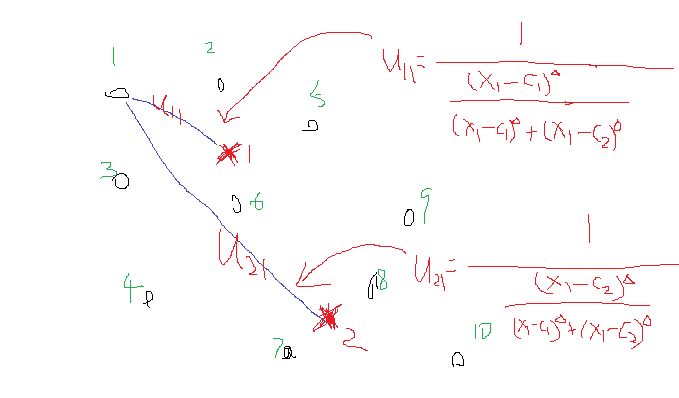再来宏观看看公式（6），考虑当类i确定后，式（6）的分母求和其实是一个常数，那么式（6）可以写成：
ci=∑j=1n(xjumij)∑j=1numij=∑j=1numij∑j=1numijxj${c}_{i}=\frac{\sum _{j=1}^{n}\left({x}_{j}{u}_{ij}^{m}\right)}{\sum _{j=1}^{n}{u}_{ij}^{m}}=\sum _{j=1}^{n}\frac{{u}_{ij}^{m}}{\sum _{j=1}^{n}{u}_{ij}^{m}}{x}_{j}$c_i=\frac{\sum\limits_{j=1}^n(x_ju_{ij}^m)}{\sum\limits_{j=1}^nu_{ij}^m}=\sum\limits_{j=1}^n\frac{u_{ij}^m}{\sum\limits_{j=1}^nu_{ij}^m}x_j
这是类中心的更新法则。说这之前，首先让我们想想kmeans的类中心是怎么更新的，一般最简单的就是找到属于某一类的所有样本点，然后这一类的类中心就是这些样本点的平均值。那么FCM类中心怎么样了？看式子可以发现也是一个加权平均，类i确定后，首先将所有点到该类的隶属度u求和，然后对每个点，隶属度除以这个和就是所占的比重，乘以xj${x}_{j}$$x_j$就是这个点对于这个类i的贡献值了。画个形象的图如下：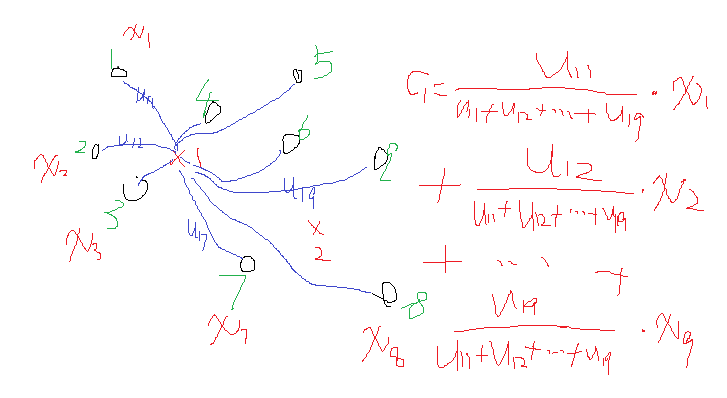由上述的宏观分析可知，这两个公式的迭代关系式是这样的也是可以理解的。

（二）简单程序实现

下面我们在matlab下用最基础的循环实现上述的式（5）与式（6）的FCM过程。首先，我们需要产生可用于FCM的数据，为了可视化方便，我们产生一个二维数据便于在坐标轴上显示，也就是每个样本由两个特征（或者x坐标与y坐标构成），生成100个这样的点，当然我们在人为改变一下，让这些点看起来至少属于不同的类。生成的点画出来如下：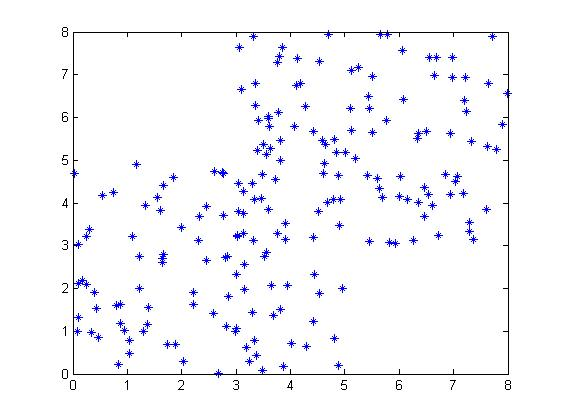那么我们说FCM算法的一般步骤为：
（1）确定分类数，指数m的值，确定迭代次数（这是结束的条件，当然结束的条件可以有多种）。
（2）初始化一个隶属度U（注意条件—和为1）；
（3）根据U计算聚类中心C；
（4）这个时候可以计算目标函数J了
（5）根据C返回去计算U，回到步骤3，一直循环直到结束。

还需要说一点的是，当程序结束后，怎么去判断哪个点属于哪个类呢？在结束后，肯定有最后一次计算的U吧，对于每一个点，它属于各个类都会有一个u，那么找到其中的最大的u就认为这个点就属于这一类。基于此一个基础的程序如下：

clc
clear
close all
%% create samples:
for i=1:100
x1(i) = rand()*5;      %人为保证差异性
y1(i) = rand()*5;
x2(i) = rand()*5 + 3; %人为保证差异性
y2(i) = rand()*5 + 3;
end
x = [x1,x2];
y = [y1,y2];
data = [x;y];
data = data';%一般数据每一行代表一个样本
%plot(data(:,1),data(:,2),'*');  %画出来
%%---
cluster_n = 2;%类别数
iter = 50;%迭代次数
m = 2;%指数

num_data = size(data,1);%样本个数
num_d = size(data,2);%样本维度
%--初始化隶属度u，条件是每一列和为1
U = rand(cluster_n,num_data);
col_sum = sum(U);
U = U./col_sum(ones(cluster_n,1),:);
%% 循环--规定迭代次数作为结束条件
for i = 1:iter
%更新c
for j = 1:cluster_n
u_ij_m = U(j,:).^m;
sum_u_ij = sum(u_ij_m);
sum_1d = u_ij_m./sum_u_ij;
c(j,:) = u_ij_m*data./sum_u_ij;
end
%-计算目标函数J
temp1 = zeros(cluster_n,num_data);
for j = 1:cluster_n
for k = 1:num_data
temp1(j,k) = U(j,k)^m*(norm(data(k,:)-c(j,:)))^2;
end
end
J(i) = sum(sum(temp1));
%更新U
for j = 1:cluster_n
for k = 1:num_data
sum1 = 0;
for j1 = 1:cluster_n
temp = (norm(data(k,:)-c(j,:))/norm(data(k,:)-c(j1,:))).^(2/(m-1));
sum1 = sum1 + temp;
end
U(j,k) = 1./sum1;
end
end
end
figure;
subplot(1,2,1),plot(J);
[~,label] = max(U); %找到所属的类
subplot(1,2,2);
gscatter(data(:,1),data(:,2),label)

得到结果如下：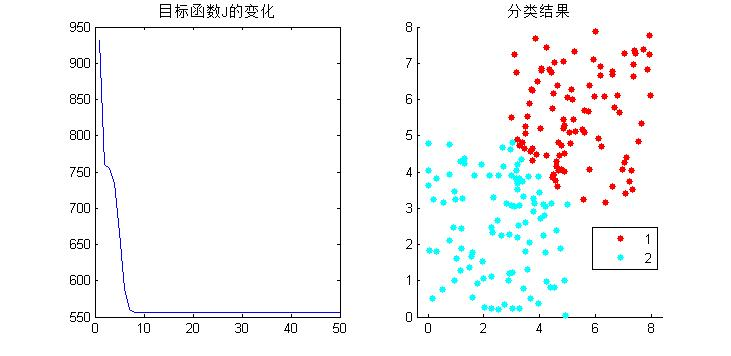分成3类看看：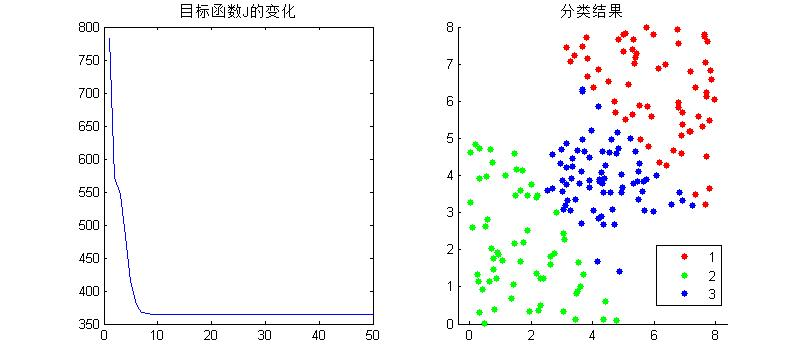基于此，结果还算正确。但是不得不说的一个问题就是算法的效率问题。为了和公式计算方式吻合，便于理解，这个程序里面有很多的循环操作，当分类数大一点，样本多一点的时候，这么写是很慢的，matlab号称矩阵实验室，所以要尽量少的使用循环，直接矩阵操作，那么上述的操作很多地方是可以把循环改成矩阵计算的，这里来介绍下matlab自带的fcm函数，就是使用矩阵运算来的。

Matlab下help fcm既可以查阅相关用法们这里只是简单介绍，fcm函数输入需要2个或者3个参数，返回3个参数，如下：
[center, U, obj_fcn] = fcm(data, cluster_n, options)
对于输入：data数据集（注意每一行代表一个样本，列是样本个数）
cluster_n为聚类数。
options是可选参数，完整的options包括：
OPTIONS(1): U的指数 (default: 2.0)
OPTIONS(2): 最大迭代次数  (default: 100)
OPTIONS(3): 目标函数的最小误差 (default: 1e-5)
OPTIONS(4): 是否显示结果  (default: 1，显示)
options都有默认值，自带的fcm结束的条件是OPTIONS(2)与OPTIONS(3)有一个满足就结束。
对于输出：center聚类中心，U隶属度，obj_fcn目标函数值，这个迭代过程的每一代的J都在这里面存着。

为了验证我们写的算法是否正确，用它的fcm去试试我们的数据（前提是数据一样），分成3类，画出它们的obj_fcn看看如下：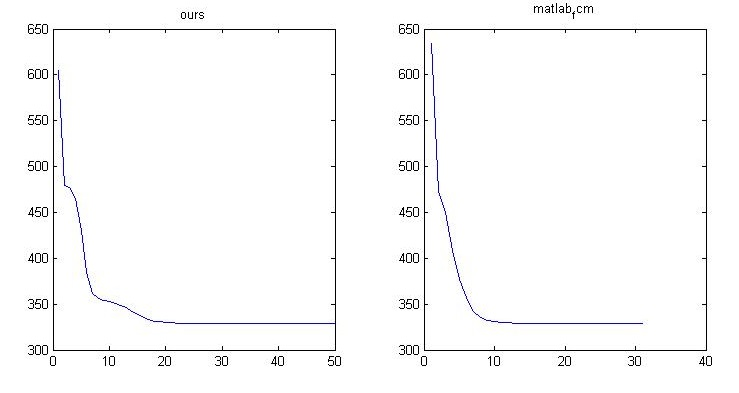可以看到，虽然迭代的中间过程不一样，但是结果却是一样的。

（三）进阶应用

了解了fcm，再来看看它的几个应用。

3.1）基于fcm的图像分割

我们知道fcm主要用于聚类，而图像分割本身就是一个聚类的过程。所以可以用fcm去实现图像分割。

这里以matlab下的灰度图像为例。灰度图像一图像的角度看是二维的，但是我们知道，决定图像的无非是里面的灰度值。而灰度值就是一个值，所以当我们把图像变成1维，也就是拉成一行或者一列的时候，其实灰度图像就是一个一维数据（上面那个例子生成的随机点是二维的）。

那么我们就可以对这个一维数据进行聚类，待得到了分类结果后，再把这个结果返回到二维图像空间去显示就可以了。
一个例子如下：

clc
clear
close all
subplot(1,2,1),imshow(img,[]);
data = img(:);
%分成4类
[center,U,obj_fcn] = fcm(data,4);
[~,label] = max(U); %找到所属的类
%变化到图像的大小
img_new = reshape(label,size(img));
subplot(1,2,2),imshow(img_new,[]);需要注意的是label出来的是标签类别（1-4），并非真实的灰度，这里不过是把它显示出来就行了。

3.2）实际数据的分类

这里介绍一个常用于机器学习、模式划分的数据下载网站：
http://archive.ics.uci.edu/ml/datasets.html

这里面包含众多的数据库可用分类划分等。这里我们选择其中一个数据库：
http://archive.ics.uci.edu/ml/datasets/seeds#

这个数据库看介绍好像是关于种子分类的，里面共包含3类种子，每类种子通过什么x射线技术等等采集他们的特征，反正最后每个种子共有7个特征值来表示它（也就是说在数据里面相当于7维），每类种子又有70个样本，那么整个数据集就是210*7的样本集。从上面那个地方下载完样本集存为txt文件，并放到matlab工作目录下就可以使用了（注意看看下下来的数据有没有串位的，有的话要手动调整回去）。因为matlab只能显示低于3维的数据，这里有7维，我们现在在二维下显示其中的两维以及正确的分类结果看看什么情况：

clc
clear
close all
data = importdata('data.txt');
%data中还有第8列，正确的标签列
subplot(2,2,1);
gscatter(data(:,1),data(:,6),data(:,8)),title('choose:1,6 列')
subplot(2,2,2);
gscatter(data(:,2),data(:,4),data(:,8)),title('choose:2,4 列')
subplot(2,2,3);
gscatter(data(:,3),data(:,5),data(:,8)),title('choose:3,5 列')
subplot(2,2,4);
gscatter(data(:,4),data(:,7),data(:,8)),title('choose:4,7 列')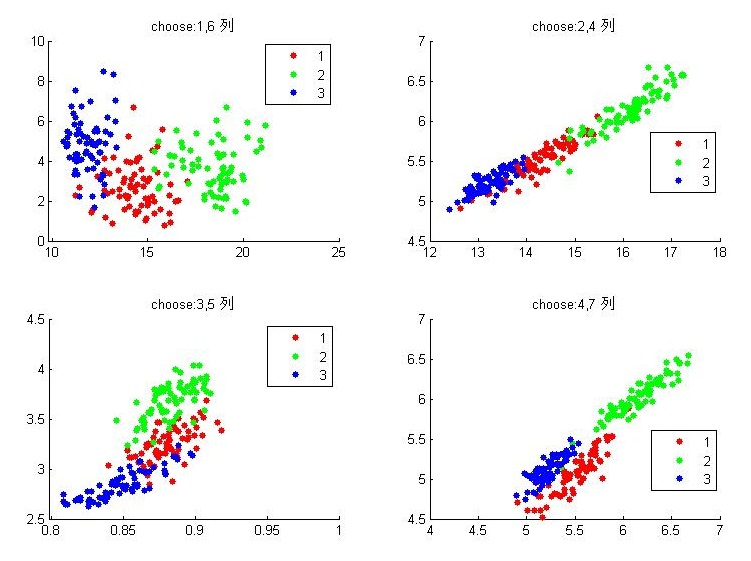组合有限，随便组合几种看看，发现似乎任意两个特征都可以把他们分开，当然还是有一些分不开的，其中最后一个选择特征4,7似乎很好的分开了。

Ok，看过之后我们来试试fcm算法对其进行分类，并计算一下准确率，我们先把7个特征都用上看看：

clc
clear
close all
data = importdata('data.txt');
%data中还有第8列，正确的标签列
[center,U,obj_fcn] = fcm(data(:,1:7),3);
[~,label] = max(U); %找到所属的类
subplot(1,2,1);
gscatter(data(:,4),data(:,7),data(:,8)),title('choose:4,7列,理论结果')
% cal accuracy
a_1 = size(find(label(1:70)==1),2);
a_2 = size(find(label(1:70)==2),2);
a_3 = size(find(label(1:70)==3),2);
a = max([a_1,a_2,a_3]);
b_1 = size(find(label(71:140)==1),2);
b_2 = size(find(label(71:140)==2),2);
b_3 = size(find(label(71:140)==3),2);
b = max([b_1,b_2,b_3]);
c_1 = size(find(label(141:210)==1),2);
c_2 = size(find(label(141:210)==2),2);
c_3 = size(find(label(141:210)==3),2);
c = max([c_1,c_2,c_3]);
accuracy = (a+b+c)/210;
subplot(1,2,2);
gscatter(data(:,4),data(:,7),label),title(['实际结果,accuracy=',num2str(accuracy)])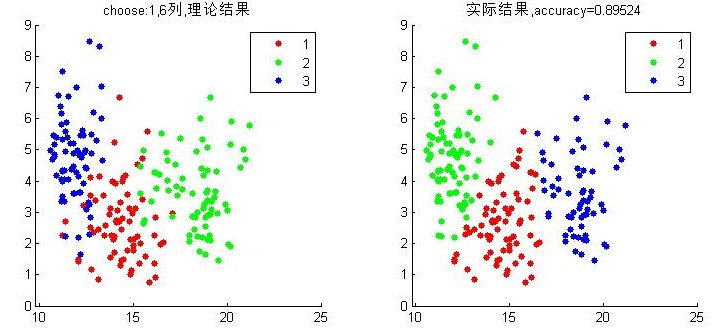这里选择以第1与6维的数据来可视化这个结果。可以看到准确率为0.89524。

这就是用了所有特征来实验的，这与用哪个特征能到达更好的结果、怎么样吧特征进行处理下能达到更好的结果，这都是机器学习与分类领域在研究的事情。上面我们感觉特征4,7不错，那么当我们只用特征4与7去进行fcm会怎样呢？好像并不是很好，想想只用特征4与7结果本来就是这样的，不好就对了，fcm是根据数据距离划分来的，所以结果就是这样。

试了很多组特征，都没有超过0.89524的，那就所有特征都用上吧。其实这个准确率是可以提高的，我们看到这7个特征似乎有点重复有没有，如果我们把这7个特征采用pca降维到3,4个特征了再去fcm实验呢？可以去试试，有待实验……
展开全文聚类 FCM 机器学习
• 模糊C均值 FCM算法是一种基于划分的聚类算法，它的思想就是使得被划分到同一簇的对象之间相似度最大，而不同簇之间的相似度最小。模糊C均值 算法是普通C均值算法的改进，普通C均值算法对于数据的划分是硬性的，而FCM...
• FCM算法是一种基于划分的聚类算法，它的思想就是使得被划分到同一簇的对象之间相似度最大，而不同簇之间的相似度最小。模糊C均值算法是普通C均值算法的改进，普通C均值算法对于数据的划分是硬性的，而FCM则是一种...
https://www.cnblogs.com/sddai/p/6259553.html

FCM算法是一种基于划分的聚类算法，它的思想就是使得被划分到同一簇的对象之间相似度最大，而不同簇之间的相似度最小。模糊C均值算法是普通C均值算法的改进，普通C均值算法对于数据的划分是硬性的，而FCM则是一种柔性的模糊划分。在介绍FCM具体算法之前我们先介绍一些模糊集合的基本知识。

1 模糊集基本知识

首先说明隶属度函数的概念。隶属度函数是表示一个对象x隶属于集合A的程度的函数，通常记做μA(x)，其自变量范围是所有可能属于集合A的对象（即集合A所在空间中的所有点），取值范围是[0,1]，即0<=μA(x)<=1。μA(x)=1表示x完全隶属于集合A，相当于传统集合概念上的x∈A。一个定义在空间X={x}上的隶属度函数就定义了一个模糊集合A，或者叫定义在论域X={x}上的模糊子集。对于有限个对象x1，x2，……，xn模糊集合可以表示为：（6.1)

有了模糊集合的概念，一个元素隶属于模糊集合就不是硬性的了，在聚类的问题中，可以把聚类生成的簇看成模糊集合，因此，每个样本点隶属于簇的隶属度就是[0，1]区间里面的值。

2 K均值聚类算法(HCM，K-Means)介绍

K均值聚类（K-Means），即众所周知的C均值聚类，已经应用到各种领域。它的核心思想如下：算法把n个向量xj(1,2…,n)分为c个组Gi(i=1,2,…,c)，并求每组的聚类中心，使得非相似性（或距离）指标的价值函数（或目标函数）达到最小。当选择欧几里德距离为组j中向量xk与相应聚类中心ci间的非相似性指标时，价值函数可定义为：(6.2)

这里是组i内的价值函数。这样Ji的值依赖于Gi的几何特性和ci的位置。

一般来说，可用一个通用距离函数d(xk,ci)代替组I中的向量xk，则相应的总价值函数可表示为：(6.3)

为简单起见，这里用欧几里德距离作为向量的非相似性指标，且总的价值函数表示为（6.2）式。

划分过的组一般用一个c×n的二维隶属矩阵U来定义。如果第j个数据点xj属于组i，则U中的元素uij为1；否则，该元素取0。一旦确定聚类中心ci，可导出如下使式（6.2）最小uij：(6.4)

重申一点，如果ci是xj的最近的聚类中心，那么xj属于组i。由于一个给定数据只能属于一个组，所以隶属矩阵U具有如下性质：(6.5)

且(6.6)

另一方面，如果固定uij则使（6.2）式最小的最佳聚类中心就是组I中所有向量的均值：(6.7)

这里|Gi|是Gi的规模或。

为便于批模式运行，这里给出数据集xi（1，2…，n）的K均值算法；该算法重复使用下列步骤，确定聚类中心ci和隶属矩阵U：

步骤1：初始化聚类中心ci,i=1,…,c。典型的做法是从所有数据点中任取c个点。

步骤2：用式（6.4）确定隶属矩阵U。

步骤3：根据式（6.2）计算价值函数。如果它小于某个确定的阀值，或它相对上次价值函数质的改变量小于某个阀值，则算法停止。

步骤4：根据式（6.5）修正聚类中心。返回步骤2。

该算法本身是迭代的，且不能确保它收敛于最优解。K均值算法的性能依赖于聚类中心的初始位置。所以，为了使它可取，要么用一些前端方法求好的初始聚类中心；要么每次用不同的初始聚类中心，将该算法运行多次。此外，上述算法仅仅是一种具有代表性的方法；我们还可以先初始化一个任意的隶属矩阵，然后再执行迭代过程。

K均值算法也可以在线方式运行。这时，通过时间平均，导出相应的聚类中心和相应的组。即对于给定的数据点x，该算法求最近的聚类中心ci，并用下面公式进行修正：(6.8)

这种在线公式本质上嵌入了许多非监督学习神经元网络的学习法则。

3   模糊C均值聚类

模糊C均值聚类（FCM），即众所周知的模糊ISODATA，是用隶属度确定每个数据点属于某个聚类的程度的一种聚类算法。1973年，Bezdek提出了该算法，作为早期硬C均值聚类（HCM）方法的一种改进。

FCM把n个向量xi（i=1,2,…,n）分为c个模糊组，并求每组的聚类中心，使得非相似性指标的价值函数达到最小。FCM与HCM的主要区别在于FCM用模糊划分，使得每个给定数据点用值在0，1间的隶属度来确定其属于各个组的程度。与引入模糊划分相适应，隶属矩阵U允许有取值在0，1间的元素。不过，加上归一化规定，一个数据集的隶属度的和总等于1：(6.9)

那么，FCM的价值函数（或目标函数）就是式（6.2）的一般化形式：(6.10)

这里uij介于0，1间；ci为模糊组I的聚类中心，dij=||ci-xj||为第I个聚类中心与第j个数据点间的欧几里德距离；且是一个加权指数。

构造如下新的目标函数，可求得使（6.10）式达到最小值的必要条件：(6.11)

这里lj，j=1到n，是（6.9）式的n个约束式的拉格朗日乘子。对所有输入参量求导，使式（6.10）达到最小的必要条件为：(6.12)

和(6.13)

由上述两个必要条件，模糊C均值聚类算法是一个简单的迭代过程。在批处理方式运行时，FCM用下列步骤确定聚类中心ci和隶属矩阵U：

步骤1：用值在0，1间的随机数初始化隶属矩阵U，使其满足式（6.9）中的约束条件

步骤2：用式（6.12）计算c个聚类中心ci，i=1,…,c。

步骤3：根据式（6.10）计算价值函数。如果它小于某个确定的阀值，或它相对上次价值函数值的改变量小于某个阀值，则算法停止。

步骤4：用（6.13）计算新的U矩阵。返回步骤2。

上述算法也可以先初始化聚类中心，然后再执行迭代过程。由于不能确保FCM收敛于一个最优解。算法的性能依赖于初始聚类中心。因此，我们要么用另外的快速算法确定初始聚类中心，要么每次用不同的初始聚类中心启动该算法，多次运行FCM。

4 FCM算法的应用

通过上面的讨论，我们不难看出FCM算法需要两个参数一个是聚类数目C，另一个是参数m。一般来讲C要远远小于聚类样本的总个数，同时要保证C>1。对于m，它是一个控制算法的柔性的参数，如果m过大，则聚类效果会很次，而如果m过小则算法会接近HCM聚类算法。

算法的输出是C个聚类中心点向量和C*N的一个模糊划分矩阵，这个矩阵表示的是每个样本点属于每个类的隶属度。根据这个划分矩阵按照模糊集合中的最大隶属原则就能够确定每个样本点归为哪个类。聚类中心表示的是每个类的平均特征，可以认为是这个类的代表点。

从算法的推导过程中我们不难看出，算法对于满足正态分布的数据聚类效果会很好，另外，算法对孤立点是敏感的。
展开全文FCM聚类算法
• ﻿﻿ ...本文关于FCM算法的一些原理推导部分介绍等参考下面视频，加上自己的理解以文字的形式呈现出来，视频参考如下，比较长，看不懂的可以再去看看： FCM原理介绍 FCM分析1 FCM分析2 FCM
﻿﻿

（一）原理部分
模糊C均值（Fuzzy C-means）算法简称FCM算法，是一种基于目标函数的模糊聚类算法，主要用于数据的聚类分析。理论成熟，应用广泛，是一种优秀的聚类算法。本文关于FCM算法的一些原理推导部分介绍等参考下面视频，加上自己的理解以文字的形式呈现出来，视频参考如下，比较长，看不懂的可以再去看看：
FCM原理介绍
FCM分析1

FCM分析2
FCM分析3
首先介绍一下模糊这个概念，所谓模糊就是不确定，确定性的东西是什么那就是什么，而不确定性的东西就说很像什么。比如说把20岁作为年轻不年轻的标准，那么一个人21岁按照确定性的划分就属于不年轻，而我们印象中的观念是21岁也很年轻，这个时候可以模糊一下，认为21岁有0.9分像年轻，有0.1分像不年轻，这里0.9与0.1不是概率，而是一种相似的程度，把这种一个样本属于结果的这种相似的程度称为样本的隶属度，一般用u表示，表示一个样本相似于不同结果的一个程度指标。
基于此，假定数据集为X，如果把这些数据划分成c类的话，那么对应的就有c个类中心为C，每个样本j属于某一类i的隶属度为uij，那么定义一个FCM目标函数（1）及其约束条件（2）如下所示：

J=∑i=1c∑j=1numij||xj−ci||2(1)

∑i=1cuij=1,j=1,2...,n(2)

看一下目标函数（式1）而知，由相应样本的隶属度与该样本到各个类中心的距离相乘组成的，m是一个隶属度的因子，个人理解为属于样本的轻缓程度，就像x2与x3这种一样。式（2）为约束条件，也就是一个样本属于所有类的隶属度之和要为1。观察式（1）可以发现，其中的变量有uij、ci，并且还有约束条件，那么如何求这个目标函数的极值呢？

这里首先采用拉格朗日乘数法将约束条件拿到目标函数中去，前面加上系数，并把式（2）的所有j展开，那么式（1）变成下列所示：

J=∑i=1c∑j=1numij||xj−ci||2+λ1(∑i=1cui1−1)+...+λj(∑i=1cuij−1)+...+λn(∑i=ncuin−1))(3)

现在要求该式的目标函数极值，那么分别对其中的变量uij、ci求导数，首先对uij求导。

分析式(3)，先对第一部分的两级求和的uij求导，对求和形式下如果直接求导不熟悉，可以把求和展开如下：

⎡⎣⎢⎢⎢⎢⎢⎢⎢⎢⎢⎢um11||x1−c1||2⋮umi1||x1−ci||2⋮umc1||x1−cc||2⋯⋮⋯⋮⋯um1j||xj−c1||2⋮umij||xj−ci||2⋮umcj||xj−cc||2⋯⋮⋯⋮⋯um1n||xn−c1||2⋮umin||xn−ci||2⋮umcn||xn−cc||2⎤⎦⎥⎥⎥⎥⎥⎥⎥⎥⎥⎥

这个矩阵要对uij求导，可以看到只有uij对应的umij||xj−ci||2保留，其他的所有项中因为不含有uij，所以求导都为0。那么umij||xj−ci||2对uij求导后就为m||xj−ci||2um−1ij。

再来看后面那个对uij求导，同样把求和展开，再去除和uij不相关的（求导为0），那么只剩下这一项：λj(uij−1)，它对uij求导就是λj了。
那么最终J对uij的求导结果并让其等于0就是：

∂J∂uij=m||xj−ci||2um−1ij+λj=0

这个式子化简下，将uij解出来就是：

um−1ij=−λjm||xj−ci||2

进一步：
uij=(−λjm||xj−ci||2)1m−1=(−λjm)1m−1(1||xj−ci||(2m−1))(4)

要解出uij则需要把λj去掉才行。这里重新使用公式（2）的约束条件，并把算出来的uij代入式（2）中有：
1=∑i=1cuij=∑i=1c(−λjm)1m−1(1||xj−ci||(2m−1))=(−λjm)1m−1∑i=1c(1||xj−ci||(2m−1))
这样就有（其中把符号i换成k）：
(−λjm)1m−1=(1∑i=1c(1||xj−ci||(2m−1)))=(1∑k=1c(1||xj−ck||(2m−1)))
把这个重新代入到式（4）中有：
uij=(1∑k=1c(1||xj−ck||(2m−1)))(1||xj−ci||(2m−1))=(1∑k=1c(||xj−ci||(2m−1))||xj−ck||(2m−1)))=1∑k=1c(||xj−ci||||xj−ck||)(2m−1)(5)
好了，式子（5）就是最终的uij迭代公式。
下面在来求J对ci的导数。由公式（2）可以看到只有∑i=1c∑j=1numij||xj−ci||2这一部分里面含有ci，对其二级求和展开如前面所示的，那么它对ci的导数就是：

∂J∂ci=∑j=1n(−umij∗2∗(xj−ci))=0

即：
∑j=1n(umijci)=∑j=1n(xjumij)
ci=∑j=1n(xjumij)∑j=1numij(6)

好了，公式（6）就是类中心的迭代公式。
我们发现uij与ci是相互关联的，彼此包含对方，有一个问题就是在fcm算法开始的时候既没有uij也没有ci，那要怎么求解呢？很简单，程序开始的时候我们随便赋值给uij或者ci其中的一个，只要数值满足条件即可。然后就开始迭代，比如一般的都赋值给uij，那么有了uij就可以计算ci，然后有了ci又可以计算uij，反反复复，在这个过程中还有一个目标函数J一直在变化，逐渐趋向稳定值。那么当J不在变化的时候就认为算法收敛到一个比较好的结了。可以看到uij和ci在目标函数J下似乎构成了一个正反馈一样，这一点很像EM算法，先E在M，有了M在E，在M直至达到最优。
公式（5），（6）是算法的关键。现在来重新从宏观的角度来整体看看这两个公式，先看（5），在写一遍

uij=1∑k=1c(||xj−ci||||xj−ck||)(2m−1)

假设看样本集中的样本1到各个类中心的隶属度，那么此时j=1，i从1到c类，此时上述式中分母里面求和中，分子就是这个点相对于某一类的类中心距离，而分母是这个点相对于所有类的类中心的距离求和，那么它们两相除表示什么，是不是表示这个点到某个类中心在这个点到所有类中心的距离和的比重。当求和里面的分子越小，是不是说就越接近于这个类，那么整体这个分数就越大，也就是对应的uij就越大，表示越属于这个类，形象的图如下：再来宏观看看公式（6），考虑当类i确定后，式（6）的分母求和其实是一个常数，那么式（6）可以写成：

ci=∑j=1n(xjumij)∑j=1numij=∑j=1numij∑j=1numijxj

这是类中心的更新法则。说这之前，首先让我们想想kmeans的类中心是怎么更新的，一般最简单的就是找到属于某一类的所有样本点，然后这一类的类中心就是这些样本点的平均值。那么FCM类中心怎么样了？看式子可以发现也是一个加权平均，类i确定后，首先将所有点到该类的隶属度u求和，然后对每个点，隶属度除以这个和就是所占的比重，乘以xj就是这个点对于这个类i的贡献值了。画个形象的图如下：由上述的宏观分析可知，这两个公式的迭代关系式是这样的也是可以理解的。

（二）简单程序实现
下面我们在matlab下用最基础的循环实现上述的式（5）与式（6）的FCM过程。首先，我们需要产生可用于FCM的数据，为了可视化方便，我们产生一个二维数据便于在坐标轴上显示，也就是每个样本由两个特征（或者x坐标与y坐标构成），生成100个这样的点，当然我们在人为改变一下，让这些点看起来至少属于不同的类。生成的点画出来如下：那么我们说FCM算法的一般步骤为：
（1）确定分类数，指数m的值，确定迭代次数（这是结束的条件，当然结束的条件可以有多种）。
（2）初始化一个隶属度U（注意条件—和为1）；
（3）根据U计算聚类中心C；
（4）这个时候可以计算目标函数J了
（5）根据C返回去计算U，回到步骤3，一直循环直到结束。
还需要说一点的是，当程序结束后，怎么去判断哪个点属于哪个类呢？在结束后，肯定有最后一次计算的U吧，对于每一个点，它属于各个类都会有一个u，那么找到其中的最大的u就认为这个点就属于这一类。基于此一个基础的程序如下：
clc
clear
close all
%% create samples:
for i=1:100
x1(i) = rand()*5;      %人为保证差异性
y1(i) = rand()*5;
x2(i) = rand()*5 + 3; %人为保证差异性
y2(i) = rand()*5 + 3;
end
x = [x1,x2];
y = [y1,y2];
data = [x;y];
data = data';%一般数据每一行代表一个样本
%plot(data(:,1),data(:,2),'*');  %画出来
%%---
cluster_n = 2;%类别数
iter = 50;%迭代次数
m = 2;%指数

num_data = size(data,1);%样本个数
num_d = size(data,2);%样本维度
%--初始化隶属度u，条件是每一列和为1
U = rand(cluster_n,num_data);
col_sum = sum(U);
U = U./col_sum(ones(cluster_n,1),:);
%% 循环--规定迭代次数作为结束条件
for i = 1:iter
%更新c
for j = 1:cluster_n
u_ij_m = U(j,:).^m;
sum_u_ij = sum(u_ij_m);
sum_1d = u_ij_m./sum_u_ij;
c(j,:) = u_ij_m*data./sum_u_ij;
end
%-计算目标函数J
temp1 = zeros(cluster_n,num_data);
for j = 1:cluster_n
for k = 1:num_data
temp1(j,k) = U(j,k)^m*(norm(data(k,:)-c(j,:)))^2;
end
end
J(i) = sum(sum(temp1));
%更新U
for j = 1:cluster_n
for k = 1:num_data
sum1 = 0;
for j1 = 1:cluster_n
temp = (norm(data(k,:)-c(j,:))/norm(data(k,:)-c(j1,:))).^(2/(m-1));
sum1 = sum1 + temp;
end
U(j,k) = 1./sum1;
end
end
end
figure;
subplot(1,2,1),plot(J);
[~,label] = max(U); %找到所属的类
subplot(1,2,2);
gscatter(data(:,1),data(:,2),label)
得到结果如下：分成3类看看：基于此，结果还算正确。但是不得不说的一个问题就是算法的效率问题。为了和公式计算方式吻合，便于理解，这个程序里面有很多的循环操作，当分类数大一点，样本多一点的时候，这么写是很慢的，matlab号称矩阵实验室，所以要尽量少的使用循环，直接矩阵操作，那么上述的操作很多地方是可以把循环改成矩阵计算的，这里来介绍下matlab自带的fcm函数，就是使用矩阵运算来的。
Matlab下help fcm既可以查阅相关用法们这里只是简单介绍，fcm函数输入需要2个或者3个参数，返回3个参数，如下：
[center, U, obj_fcn] = fcm(data, cluster_n, options)
对于输入：data数据集（注意每一行代表一个样本，列是样本个数）
cluster_n为聚类数。
options是可选参数，完整的options包括：
OPTIONS(1): U的指数 (default: 2.0)
OPTIONS(2): 最大迭代次数 (default: 100)
OPTIONS(3): 目标函数的最小误差 (default: 1e-5)
OPTIONS(4): 是否显示结果 (default: 1，显示)
options都有默认值，自带的fcm结束的条件是OPTIONS(2)与OPTIONS(3)有一个满足就结束。
对于输出：center聚类中心，U隶属度，obj_fcn目标函数值，这个迭代过程的每一代的J都在这里面存着。
为了验证我们写的算法是否正确，用它的fcm去试试我们的数据（前提是数据一样），分成3类，画出它们的obj_fcn看看如下：可以看到，虽然迭代的中间过程不一样，但是结果却是一样的。
（三）进阶应用
了解了fcm，再来看看它的几个应用。
3.1）基于fcm的图像分割
我们知道fcm主要用于聚类，而图像分割本身就是一个聚类的过程。所以可以用fcm去实现图像分割。
这里以matlab下的灰度图像为例。灰度图像一图像的角度看是二维的，但是我们知道，决定图像的无非是里面的灰度值。而灰度值就是一个值，所以当我们把图像变成1维，也就是拉成一行或者一列的时候，其实灰度图像就是一个一维数据（上面那个例子生成的随机点是二维的）。
那么我们就可以对这个一维数据进行聚类，待得到了分类结果后，再把这个结果返回到二维图像空间去显示就可以了。
一个例子如下：
clc
clear
close all
subplot(1,2,1),imshow(img,[]);
data = img(:);
%分成4类
[center,U,obj_fcn] = fcm(data,4);
[~,label] = max(U); %找到所属的类
%变化到图像的大小
img_new = reshape(label,size(img));
subplot(1,2,2),imshow(img_new,[]);需要注意的是label出来的是标签类别（1-4），并非真实的灰度，这里不过是把它显示出来就行了。
3.2）实际数据的分类
这里介绍一个常用于机器学习、模式划分的数据下载网站：
http://archive.ics.uci.edu/ml/datasets.html
这里面包含众多的数据库可用分类划分等。这里我们选择其中一个数据库：
http://archive.ics.uci.edu/ml/datasets/seeds#
这个数据库看介绍好像是关于种子分类的，里面共包含3类种子，每类种子通过什么x射线技术等等采集他们的特征，反正最后每个种子共有7个特征值来表示它（也就是说在数据里面相当于7维），每类种子又有70个样本，那么整个数据集就是210*7的样本集。从上面那个地方下载完样本集存为txt文件，并放到matlab工作目录下就可以使用了（注意看看下下来的数据有没有串位的，有的话要手动调整回去）。因为matlab只能显示低于3维的数据，这里有7维，我们现在在二维下显示其中的两维以及正确的分类结果看看什么情况：
clc
clear
close all
data = importdata('data.txt');
%data中还有第8列，正确的标签列
subplot(2,2,1);
gscatter(data(:,1),data(:,6),data(:,8)),title('choose:1,6 列')
subplot(2,2,2);
gscatter(data(:,2),data(:,4),data(:,8)),title('choose:2,4 列')
subplot(2,2,3);
gscatter(data(:,3),data(:,5),data(:,8)),title('choose:3,5 列')
subplot(2,2,4);
gscatter(data(:,4),data(:,7),data(:,8)),title('choose:4,7 列')组合有限，随便组合几种看看，发现似乎任意两个特征都可以把他们分开，当然还是有一些分不开的，其中最后一个选择特征4,7似乎很好的分开了。
Ok，看过之后我们来试试fcm算法对其进行分类，并计算一下准确率，我们先把7个特征都用上看看：
clc
clear
close all
data = importdata('data.txt');
%data中还有第8列，正确的标签列
[center,U,obj_fcn] = fcm(data(:,1:7),3);
[~,label] = max(U); %找到所属的类
subplot(1,2,1);
gscatter(data(:,4),data(:,7),data(:,8)),title('choose:4,7列,理论结果')
% cal accuracy
a_1 = size(find(label(1:70)==1),2);
a_2 = size(find(label(1:70)==2),2);
a_3 = size(find(label(1:70)==3),2);
a = max([a_1,a_2,a_3]);
b_1 = size(find(label(71:140)==1),2);
b_2 = size(find(label(71:140)==2),2);
b_3 = size(find(label(71:140)==3),2);
b = max([b_1,b_2,b_3]);
c_1 = size(find(label(141:210)==1),2);
c_2 = size(find(label(141:210)==2),2);
c_3 = size(find(label(141:210)==3),2);
c = max([c_1,c_2,c_3]);
accuracy = (a+b+c)/210;
subplot(1,2,2);
gscatter(data(:,4),data(:,7),label),title(['实际结果,accuracy=',num2str(accuracy)])这里选择以第1与6维的数据来可视化这个结果。可以看到准确率为0.89524。
这就是用了所有特征来实验的，这与用哪个特征能到达更好的结果、怎么样吧特征进行处理下能达到更好的结果，这都是机器学习与分类领域在研究的事情。上面我们感觉特征4,7不错，那么当我们只用特征4与7去进行fcm会怎样呢？好像并不是很好，想想只用特征4与7结果本来就是这样的，不好就对了，fcm是根据数据距离划分来的，所以结果就是这样。
试了很多组特征，都没有超过0.89524的，那就所有特征都用上吧。其实这个准确率是可以提高的，我们看到这7个特征似乎有点重复有没有，如果我们把这7个特征采用pca降维到3,4个特征了再去fcm实验呢？


展开全文• (1),FCM原理部分详解和代码部分（matlap编写的）： https://blog.csdn.net/on2way/article/details/47087201 （2）python实现 模糊C均值聚类算法（Fuzzy-C-Means）-基于iris数据集 ... （2）拉格朗日数乘...
(1),FCM原理部分详解和代码部分（matlap编写的）：

https://blog.csdn.net/on2way/article/details/47087201

（2）python实现 模糊C均值聚类算法（Fuzzy-C-Means）-基于iris数据集

https://blog.csdn.net/zwqhehe/article/details/75174918

（2）拉格朗日数乘法：

https://blog.csdn.net/acdreamers/article/details/41413445

说明：以上转载整理是为了方便自己回顾与查阅，
展开全文•fcm 详解
FCM，即Firebase Cloud Messaging

一、FCM初认识

我们知道，当我们应用被强杀之后，基本上就是收不到推送消息啦，或者说叫离线推送。不管是极光，腾讯信鸽还是阿里推送等，都是基本上对强杀无解，虽然第三方推送有各种奇技淫巧，但是只是杯水车薪。

而iOS在离线消息十分靠谱，那是因为每一个苹果可以通过自家服务器维持一个长链接，每一个iOS的推送都必须和苹果打交道，所以这个过程控制得很好。

GCM (Google Cloud Message for Android)是Google发布的Android服务器推送（push）技术。
FCM是谷歌推出的最新的Android系统级别的消息推送服务（用来替换GCM）。
二、接入FCM

二.1、前提

想要使用FCM，需要满足如下几个条件

Android SDK Manager 必须有Google Play services SDK
Android Studio必须是1.5以上版本

二.2、 接入

什么都别说啦，赶紧看一下Firebase FCM接入文档

本文是按照文档接入测试的，你可以自己去看啊，没错啊，到这里你就可以选择关闭页面，如果你愿意看，那么，continue。

二.2.1、在Firebase控制台添加自己的应用

创建应用image.png

.
选择添加到安卓应用image.png

.image.png

.image.png

.

二.2.2、添加使用Firebase SDK

添加SDK

buildscript → dependenciesclasspath 'com.google.gms:google-services:3.1.0'
(最新版本可根据官网文档)

allprojects → repositoriesmaven { url 'https://maven.google.com' }

这点官网无涉及，但是没加可能会报异常image.png

.
.

dependencies
    compile 'com.google.firebase:firebase-messaging:11.2.0'


.

在文件的最后添加apply plugin: 'com.google.gms.google-services'

这样代码不要有洁癖放在顶部，想着所有的apply plugin都放一起，不然很大可能报大概如下错误

Please fix the version conflict either by updating the version of the google-services plugin (information about the latest version is available at https://bintray.com/android/android-tools/com.google.gms.google-services/) or updating the version of com.google.android.gms to 9.0.0.


.image.png

题外话

    // 下方的firebase-messaging 和 play-services-maps 和 play-services版本号必须一致


配置FireBase SDK

唯一Token的获取和上传
最初启动您的应用时，FCM SDK 会为客户端应用实例生成一个注册令牌。如果您希望定位单台设备或创建设备组，则需要通过继承 FirebaseInstanceIdService
来访问此令牌。

当您需要检索当前令牌时，请调用 FirebaseInstanceId.getInstance().getToken()
如果令牌尚未生成，此方法将返回 null。

.
.消息的接收监听

MyFirebaseMessagingService
/**
* User: LJM
* Date&Time: 2017-08-25 & 10:50
* Describe: 消息接收服务
*/
public class MyFirebaseMessagingService extends FirebaseMessagingService {

private static final String TAG = "FBTEST";

@Override

Log.d(TAG, "收到推送 From: " + remoteMessage.getFrom());

// Check if message contains a data payload.
if (remoteMessage.getData().size() > 0) {
Log.d(TAG, "收到推送 Message data payload: " + remoteMessage.getData());
}

}
}

}


.
.token变化的监听

MyFirebaseInstanceIDService
/**
* User: LJM
* Date&Time: 2017-08-25 & 10:48
* Describe: 实例化令牌服务
* 处理创建，轮询更新Token，当我们推送到执行设备或者设备组的时候就需要用到这个
*/
public class MyFirebaseInstanceIDService extends FirebaseInstanceIdService {

private static final String TAG = "FBTEST";

@Override
public void onTokenRefresh() {
super.onTokenRefresh();
String refreshedToken = FirebaseInstanceId.getInstance().getToken();
Log.d(TAG, "刷新Token Refreshed token: " + refreshedToken);

// If you want to send messages to this application instance or
// manage this apps subscriptions on the server side, send the
// Instance ID token to your app server.
sendRegistrationToServer(refreshedToken);
}

/**
* Persist token to third-party servers.
*
* Modify this method to associate the user's FCM InstanceID token with any server-side account
*
* @param token The new token.
*/
private void sendRegistrationToServer(String token) {
// TODO: Implement this method to send token to your app server.
}

}


注册令牌可能会在发生下列情况时更改：

应用删除实例 ID
应用在新设备上恢复
用户卸载/重新安装应用
用户清除应用数据。
所以Firebase强烈建议我们复写onTokenRefresh方法并且及时上传更新的Token通知自家服务器

注册service
.清单文件注册service


<service android:name=".service.MyFirebaseInstanceIDService">
<intent-filter>
</intent-filter>
</service>

<service
android:name=".service.MyFirebaseMessagingService">
<intent-filter>
</intent-filter>
</service>

<!--指定图标 可选-->
<meta-data
android:resource="@drawable/shownf" />

<!--指定颜色 可选-->
<meta-data
android:resource="@color/colorPrimary" />


.
.
至此，配置完成，就等着接消息啦。

三、接收推送

（dataMessage必须服务器代码发）控制台

.image.png

发送即可收到。

经测试，Pixel 运行Oreo 完美接收，但是如果是其他厂商的机型，想要强杀后继续收到离线消息，一般需要在设置一下

小米【MIUI】

自启动管理：需要把应用加到【自启动管理】列表，否则杀进程或重新开机后进程无法开启
通知栏设置：应用默认都是显示通知栏通知，如果关闭，则收到通知也不会提示
网络助手：可以手动禁止已安装的第三方程序访问2G/3G和WIFI的网络和设置以后新安装程序是否允许访问2G/3G和WIFI的网络
MIUI 7 神隐模式： 允许应用进行自定义配置模式，应用在后台保持联网可用，否则应用进入后台时，应用无法正常接收消息。【设置】下电量和性能中【神隐模式】

华为【Emotion】

自启动管理：需要把应用加到【自启动管理】列表，否则杀进程或重新开机后进程不会开启，只能手动开启应用
后台应用保护：需要手动把应用加到此列表，否则设备进入睡眠后会自动杀掉应用进程，只有手动开启应用才能恢复运行
通知管理：应用状态有三种：提示、允许、禁止。禁止应用则通知栏不会有任何提醒

魅族【Flyme】

自启动管理：需要把应用加到【自启动管理】列表，否则杀进程或重新开机后进程无法开启
通知栏推送：关闭应用通知则收到消息不会有任何展示
省电管理： 安全中心里设置省电模式，在【待机耗电管理】中允许应用待机时，保持允许，否则手机休眠或者应用闲置一段时间，无法正常接收消息。

VIVO【Funtouch OS】

内存一键清理：需要将应用加入【白名单】列表，否则系统自带的“一键加速”，会杀掉进程
自启动管理：需要将应用加入“i管家”中的【自启动管理】列表，否则重启手机后进程不会自启。但强制手动杀进程，即使加了这个列表中，后续进程也无法自启动。

OPPO【ColorOS】

冻结应用管理：需要将应用加入纯净后台，否则锁屏状态下无法及时收到消息
自启动管理：将应用加入【自启动管理】列表的同时，还需要到设置-应用程序-正在运行里锁定应用进程，否则杀进程或者开机后进程不会开启，只能手动开启应用

三星

内存一键优化：需要将应用加入【白名单】列表，否则系统内存优化后，会杀掉应用进程

小米【MIUI】

自启动管理：需要把应用加到【自启动管理】列表，否则杀进程或重新开机后进程无法开启
通知栏设置：应用默认都是显示通知栏通知，如果关闭，则收到通知也不会提示
网络助手：可以手动禁止已安装的第三方程序访问2G/3G和WIFI的网络和设置以后新安装程序是否允许访问2G/3G和WIFI的网络
MIUI 7 神隐模式： 允许应用进行自定义配置模式，应用在后台保持联网可用，否则应用进入后台时，应用无法正常接收消息。【设置】下电量和性能中【神隐模式】

华为【Emotion】

自启动管理：需要把应用加到【自启动管理】列表，否则杀进程或重新开机后进程不会开启，只能手动开启应用
后台应用保护：需要手动把应用加到此列表，否则设备进入睡眠后会自动杀掉应用进程，只有手动开启应用才能恢复运行
通知管理：应用状态有三种：提示、允许、禁止。禁止应用则通知栏不会有任何提醒

魅族【Flyme】

自启动管理：需要把应用加到【自启动管理】列表，否则杀进程或重新开机后进程无法开启
通知栏推送：关闭应用通知则收到消息不会有任何展示
省电管理： 安全中心里设置省电模式，在【待机耗电管理】中允许应用待机时，保持允许，否则手机休眠或者应用闲置一段时间，无法正常接收消息。

VIVO【Funtouch OS】

内存一键清理：需要将应用加入【白名单】列表，否则系统自带的“一键加速”，会杀掉进程
自启动管理：需要将应用加入“i管家”中的【自启动管理】列表，否则重启手机后进程不会自启。但强制手动杀进程，即使加了这个列表中，后续进程也无法自启动。

OPPO【ColorOS】

冻结应用管理：需要将应用加入纯净后台，否则锁屏状态下无法及时收到消息
自启动管理：将应用加入【自启动管理】列表的同时，还需要到设置-应用程序-正在运行里锁定应用进程，否则杀进程或者开机后进程不会开启，只能手动开启应用

三星

内存一键优化：需要将应用加入【白名单】列表，否则系统内存优化后，会杀掉应用进程

设置方式引自极光推送文档，侵删

对应设置后，华为荣耀7，P9，�三星等均能接收离线消息。

四、服务器

参见文档

end

转载自：https://www.jianshu.com/p/d3c6ee3ae122

展开全文FCM GCM google推送
• 第一个文件（FCMTEST.py） import numpy as np import matplotlib.pyplot as plt plt.rcParams['font.sans-serif']=['SimHei'] ...class FCM: def __init__(self, data, clust_num): self.data = datapython 聚类
• 作者：史婧羽，开发技术推广工程师，Partner DevRel本文为 Android 电量管理系列连载的第二篇，希望您能在阅读过程中收获设备续航方面的战略洞见和实践指导。...
• 伴随着模糊集理论的形成、发展和深化，RusPini率先提出模糊划分的概念。以此为起点和基础，模糊聚类理论和方法迅速蓬勃发展起来。针对不同的应用，人们提出了很多模糊聚类算法，比较典型的有基于相似性关系和模糊...
• 1.建谷歌账号 ... 3.创建测试网页应用 （或app应用） C#服务端： 用HTTP v1 API 的方式获取OAuth2的验证，然后再发http请求到FCM发送消息通知。 1.获取Token 1 public async Task<string&...
• FCM推导过程超详细 最近毕业，然后可能用到FCM，找了挺多博客论文，更多的是关于用法没有推导过程，于是自己总结写一篇详细版尽量小白也能看懂的fcm推导过程，加深对算法的理解。标题或内容可能会挺暴力，个人习惯...聚类 算法 数据挖掘 机器学习Android
• FCM聚类与K-means聚类的分析比较一、FCM聚类1.简介2.FCM聚类算法原理基本步骤流程图二、数据集介绍1.数据集来源2.详细介绍三、FCM聚类实现1.Matlab代码2.运行结果（5次）3.分析四、K-means聚类实现1.K-means算法基本...
• FCM算法FCM算法简介FCM算法原理FCM算法实现(matlab) FCM算法简介 FCM算法属于划分式聚类算法，用模糊的方法来处理聚类问题，他从一个初始划分开始，需要预先指定聚类数目，还需要定义一个最优化聚类标准，也就是目标...matlab 模糊K均值算法 FCM
• 首先FCM在图像分割领域有很多应用，FCM算法我觉得主要是两个部分，一个是模糊理论，一个是C/Kmean算法，这两者在数学家手中完美的结合。 下面切入整体，我也是为了温故才写的这篇博客，如有错误或者瑕疵的地方欢迎...FCM 模糊聚类 图像分割 matlab
• 一个朋友让帮忙做图像分类，用FCM聚类算法，网上查了一下，FCM基本都是对一幅图像进行像素的分类，跟他说的任务不太一样，所要做的是将一个文件夹里的一千多幅图像进行分类。图像大概是这个样子的（是25*25的小图像...
• 1. FCM初识  FCM的C跟K-Means的K是一样的，指的是聚类的数目。F—Fuzzy是模糊的意思，指的是”一个事件发生的程度“。用在我们的聚类上面，第一条记录以怎样的概率或者说程度属于第一类，又以怎样的程度属于第二类...
• FCM算法是一种重叠聚类算法，它计算数据集中每个数据点与分类的匹配度，近日写个python程序重温了一下FCM算法。 一、算法代码 给定同维向量数据集合points，数目为n，将其聚为C类，m为权重值，u为初始匹配度矩阵...fcm 机器学习 算法 python
• FCM是google提供的一个消息推送服务，支持IOS, ANDROID, WEB浏览器等。 推送功能： 单设备推送 主题推送(合适多设备, 好像最多1000个设备) 组推送(适合某个人的多台设备， 最多20个) 说明下：因为是google服务， ...php FCM GCM
...